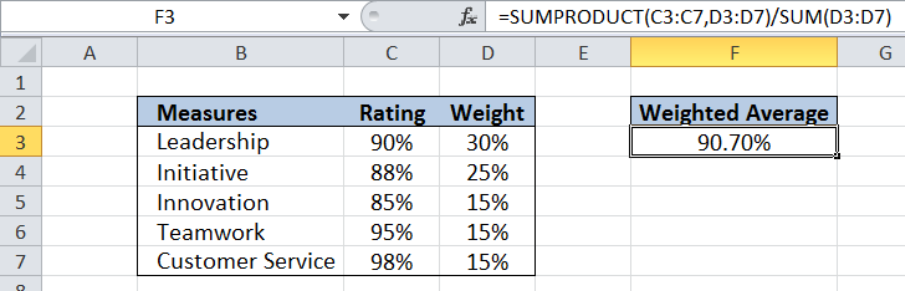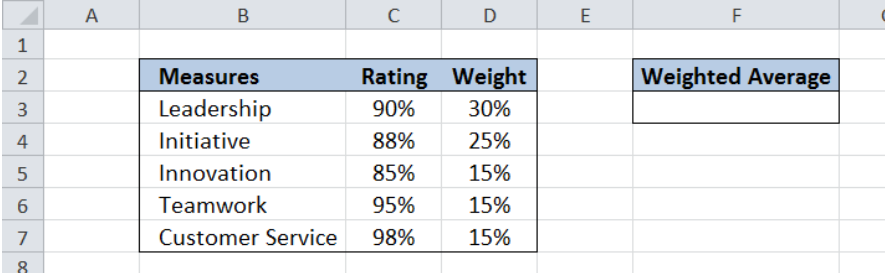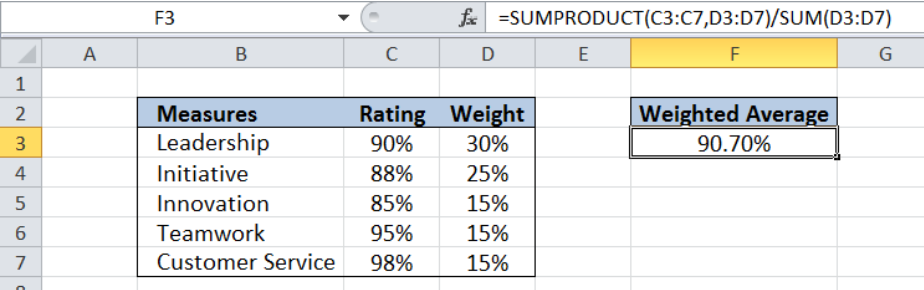Get instant live expert help with Excel or Google Sheets“My Excelchat expert helped me in less than 20 minutes, saving me what would have been 5 hours of work!”

#### Post your problem and you’ll get Expert help in seconds.

Your message must be at least 40 characters
Our professional Expert are available now. Your privacy is guaranteed.

# How to Calculate the Weighted Average in Excel

When we want to calculate the average of a set of values with different levels of relevance, we compute for the weighted average.  Excel allows us to calculate the weighted average using the SUMPRODUCT and SUM functions.Figure 1. Final result: Calculate the weighted average

Final formula: `=SUMPRODUCT(C3:C7,D3:D7)/SUM(D3:D7)`

## Syntax of the SUMPRODUCT Function

SUMPRODUCT returns the sum of the products of two or more components in the given arrays.

`=SUMPRODUCT(array1, [array2], [array3], ...)`

where

• array – a range of values or cells whose values we want to multiply with other values and then add
• All array arguments must be in the same size
• Non-numeric values in an array are treated as zeros

## Syntax of SUM Function

`=SUM(number1,[number2],...])`

• number1 – any number, array or cell reference whose values we want to add
• Only number1 is required; succeeding numbers are optional

## Setting up the Data

Our table consists of three columns: Measures (column B),  Rating (column C) and Weight (column D). In cell F3, we will calculate the weighted average of the ratings.Figure 2. Sample data to calculate the weighted average

# Calculate the weighted average of the ratings

In order to calculate the weighted average of the ratings, we follow these steps:

Step 1. Select cell F3

Step 2. Enter the formula: `=``SUMPRODUCT(C3:C7,D3:D7)/SUM(D3:D7)`

Step 3. Press Enter

The SUMPRODUCT returns the sum of the products of the ratings and weights (rating x weight).  Actual calculation of the SUMPRODUCT looks like this:

`=(C3xD3) + (C4xD4) + (C5xD5)`

The SUM function returns the total of the weights.

The SUMPRODUCT divided by the SUM function returns the value of the weighted average of the ratings.  As a result, the value in cell F3 is 90.70%.Figure 3. Using SUMPRODUCT and SUM to calculate weighted average

Most of the time, the problem you will need to solve will be more complex than a simple application of a formula or function. If you want to save hours of research and frustration, try our live Excelchat service! Our Excel Experts are available 24/7 to answer any Excel question you may have. We guarantee a connection within 30 seconds and a customized solution within 20 minutes.

Are you still looking for help with the Average function? View our comprehensive round-up of Average function tutorials here.

### Did this post not answer your question? Get a solution from connecting with the expert.Another blog reader asked this question today on Excelchat:
Solution examplesI need a formula to pop at the upper left corner of a spreadsheet. If I enter the month "January," I want the column number sum of January =SUM(AB11:AB75) from another section on the same excel page to pop right below the "January" cell, and not display the formula expression, but see the \$100.
Solved by T. Q. in 40 minsCan't add (SUM) in imported numbers from bank account
Solved by F. C. in 40 minsI need a formula to combine D2 to D100 to add together a column of numbers, then take away the same amount on the same row when column E is filled. i.e. column D is a price of an item, so the formula must calculate the total, then when the item is sold an 'a' is marked next to the item in column E, the formula then must deduct this amount from the total
Solved by X. W. in 20 minsI would like to have a diagram in a new sheet, where the horizontal axis is the days, as they are in column DX. Each day shall show the sum of all unique leads of that day, and I would like to be able to check via a box of checkboxes, which facilities are shown, the facilities are in column BC.
Solved by I. A. in 45 minsI am working on a cash flow projection. Part of the projection includes sales commissions. Our sales guys earn a monthly draw and then commission on sales after a certain amount. For example they may earn a monthly salary of 12,500 and earn additional commission after their commission equals \$150,000. What I need excel to do is sum a column if the values in the preceeding columns are greater than \$150,000. I've tried using the sumif and the if function in excel and it's not working correctly either way. Any suggestions?
Solved by G. W. in 19 mins## Subscribe to Excelchat.coAnother blog reader asked this question today on Excelchat: